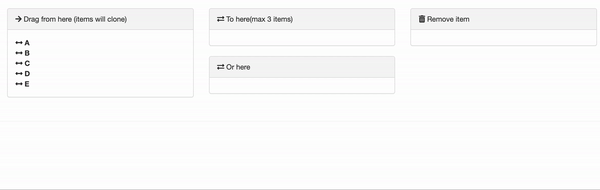# Cloning and removing elements

## Introduction

Sometimes you want the ability to drag an item multiple times from a list, in other words the ability to “clone” the items of the original list.

And you may also then want the ability to remove cloned items, possibly by dragging to a “bin” or “remove item” list.### Cloning an element

To clone an element from a list, you must add the pull = "clone" option to the sortable_options argument::

library(sortable)
sortable_js(
"sort1"
options = sortable_options(
group = list(
pull = "clone",
name = "sortGroup1",
put = FALSE
),
onSort = sortable_js_capture_input("sort_vars")
)
)

### Removing an element

To remove an element from the dropped list, one option is to create a “bin” area by using the JavaScript code:

this.el.removeChild(evt.item);

Then add to this JavaScript to the onAdd element of sortable_options(). To pass your JavaScript code to R, use the htmlwidgets::JS() function:

sortable_js(
"sortable_bin",
options = sortable_options(
group = list(
group = "sortGroup1",
put = TRUE,
pull = TRUE
),
onAdd = htmlwidgets::JS("function (evt) { this.el.removeChild(evt.item); }")
)
)

## Full example

And the full code:

## Example shiny app to demonstrate cloning and other sortable_options

library(shiny)
library(htmlwidgets)
library(sortable)
library(magrittr)

icon_list <- function(x){
lapply(
x,
function(x) {
tags$div( icon("arrows-alt-h"), tags$strong(x)
)
}
)
}

ui <- fluidPage(
fluidRow(
div(
h3("Illustration of sortable_options()")
),
fluidRow(
class = "panel-body",
column(
width = 4,
tags$div( class = "panel panel-default", tags$div(
icon("arrow-right"),
"Drag from here (items will clone)"
),
tags$div( class = "panel-body", id = "sort1", icon_list(c( "A", "B", "C", "D", "E" )) ) ) ), column( width = 4, # analyse as x tags$div(
class = "panel panel-default",
tags$div( class = "panel-heading", icon("exchange"), "To here(max 3 items)" ), tags$div(
class = "panel-body",
id = "sort2"
)
),
# analyse as y
tags$div( class = "panel panel-default", tags$div(
icon("exchange"),
"Or here"
),
tags$div( class = "panel-body", id = "sort3" ) ) ), column( width = 4, # bin tags$div(
class = "panel panel-default",
tags$div( class = "panel-heading", icon("trash"), "Remove item" ), tags$div(
class = "panel-body",
id = "sortable_bin"
)
)

)
)
),
sortable_js(
"sort1",
options = sortable_options(
group = list(
pull = "clone",
name = "sortGroup1",
put = FALSE
),
# swapClass = "sortable-swap-highlight",
onSort = sortable_js_capture_input("sort_vars")
)
),
sortable_js(
"sort2",
options = sortable_options(
group = list(
group = "sortGroup1",
pull = TRUE
),
swapClass = "sortable-swap-highlight",
onSort = sortable_js_capture_input("sort_x")
)
),
sortable_js(
"sort3",
options = sortable_options(
group = list(
group = "sortGroup1",
put = TRUE,
pull = TRUE
),
swapClass = "sortable-swap-highlight",
onSort = sortable_js_capture_input("sort_y")
)
),
sortable_js(
"sortable_bin",
options = sortable_options(
group = list(
group = "sortGroup1",
put = TRUE,
pull = TRUE
),
onAdd = htmlwidgets::JS("function (evt) { this.el.removeChild(evt.item); }")
)
)

)

server <- function(input, output) {
output$variables <- renderPrint(input[["sort_vars"]]) output$analyse_x <- renderPrint(input[["sort_x"]])
output$analyse_y <- renderPrint(input[["sort_y"]]) x <- reactive({ x <- input$sort_x
if (is.character(x)) x %>% trimws()
})

y <- reactive({
input\$sort_y %>% trimws()
})

}
shinyApp(ui, server)